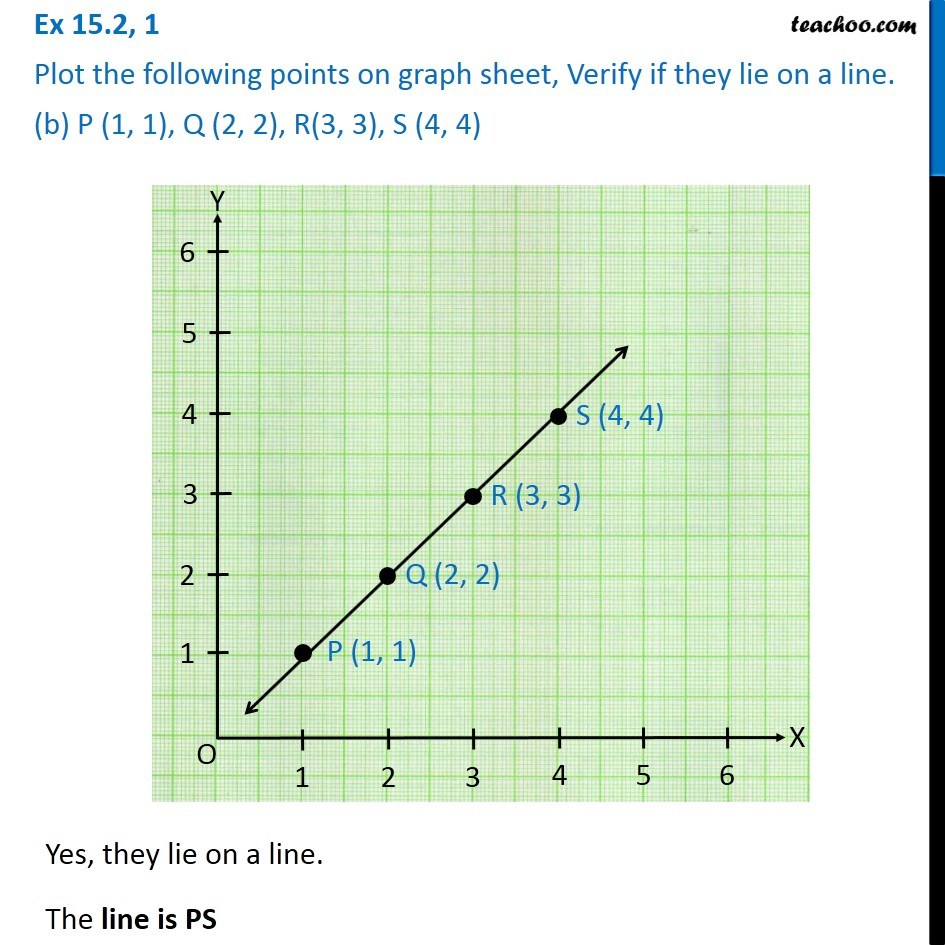1. Chapter 15 Class 8 Introduction to Graphs
2. Serial order wise
3. Ex 15.2

Transcript

Ex 15.2, 1 Plot the following points on graph sheet, Verify if they lie on a line. (b) P (1, 1), Q (2, 2), R(3, 3), S (4, 4)Yes, they lie on a line. The line is PS